[BACK]
 Computers, Materials & ContinuaDOI:10.32604/cmc.2022.019867Article

AMBO: All Members-Based Optimizer for Solving Optimization Problems

1Department of Mathematics and Computer Sciences, Sirjan University of Technology, Sirjan, Iran
2Department of Electrical and Electronics Engineering, Shiraz University of Technology, Shiraz, Iran
3Department of Mathematics, Faculty of Science, University of Hradec Králové, Hradec Králové, 50003, Czech Republic
4Department of Computer Science, Government Bikram College of Commerce, Patiala, Punjab, India
*Corresponding Author: Pavel Trojovský. Email: pavel.trojovsky@uhk.cz
Received: 29 April 2021; Accepted: 18 June 2021

Abstract: There are many optimization problems in different branches of science that should be solved using an appropriate methodology. Population-based optimization algorithms are one of the most efficient approaches to solve this type of problems. In this paper, a new optimization algorithm called All Members-Based Optimizer (AMBO) is introduced to solve various optimization problems. The main idea in designing the proposed AMBO algorithm is to use more information from the population members of the algorithm instead of just a few specific members (such as best member and worst member) to update the population matrix. Therefore, in AMBO, any member of the population can play a role in updating the population matrix. The theory of AMBO is described and then mathematically modeled for implementation on optimization problems. The performance of the proposed algorithm is evaluated on a set of twenty-three standard objective functions, which belong to three different categories: unimodal, high-dimensional multimodal, and fixed-dimensional multimodal functions. In order to analyze and compare the optimization results for the mentioned objective functions obtained by AMBO, eight other well-known algorithms have been also implemented. The optimization results demonstrate the ability of AMBO to solve various optimization problems. Also, comparison and analysis of the results show that AMBO is superior and more competitive than the other mentioned algorithms in providing suitable solution.

Keywords: Algorithm; all members; optimization; optimization algorithm; optimization problem; population-based algorithm

1  Introduction

Optimization is defined as finding the best solution out of all possible solutions to a problem by considering the constraints and limitations. Therefore, each optimization problem consists of three main parts: decision variables, primary objectives, and secondary objectives. The decision variables are the same as the problem variables, the primary objectives represent the constraints of the problem, and the secondary objectives are the objective functions of the problem.

Population-based optimization algorithms (PBOAs) are one of the most effective methods for solving optimization problems. PBOAs are able to provide appropriate solutions to optimization problems based on random scan of the search space and through an iterative-based process . Each optimization problem has a best basic solution called global optimum. On the other hand, the solution obtained by PBOAs for the optimization problem is not necessarily the global optimal solution. Hence, the solution provided by PBOAs is referred as quasi-optimal solution [2,3]. Whatever the quasi-optimal solution provided by an algorithm is closer to the global optimum, that algorithm has a better performance in solving that optimization problem. For this reason, various PBOAs have been introduced by scientists to provide quasi-optimal solutions to optimization problems. In this regard, optimization algorithms have been applied in various fields in the literature such as energy , protection , electrical engineering , and energy carriers [15,16] to achieve the optimal solution.

PBOAs are designed based on simulation of various natural phenomena, behavior of living organisms, physical laws, genetic sciences, rules of the games, and so on. In a general classification based on the design idea, PBOAs are categorized into 4 groups: swarm-based, physics-based, evolutionary-based, and game-based optimization algorithms.

Swarm-based optimization algorithms are designed based on simulating the behavior of living organisms such as animals, plants, and natural phenomena. Particle Swarm Optimization (PSO) is one of the most widely-used algorithms in this category, which is based on simulating behaviors of birds’ swarm . Ant Colony Optimization (ACO) is another swarm-based optimization technique that has been introduced based on the behavior of ants in finding the shortest route between their nest and the food source . Simulation of the patient treatment process by the doctor has been used in designing doctor and patient optimizer (DPO) . Some of the other swarm-based optimization algorithms are: Seagull Optimization Algorithm (SOA) , Whale Optimization Algorithm (WOA) , Firefly Algorithm (FA) , Artificial Bee Colony (ABC) , Cuckoo Search (CS) , Bat-inspired Algorithm (BA) , Spotted Hyena Optimizer (SHO) , Monkey Search (MS) , Artificial Fish-Swarm Algorithm (AFSA) , Group Optimization (GO) , Dolphin Partner Optimization (DPO) , Hunting Search (HS) , Coupled Spring Forced Bat Algorithm (SFBA) , Teaching-Learning-Based Optimization (TLBO) , Grey Wolf Optimizer (GWO) , Following Optimization Algorithm (FOA) , Moth-Flame Optimization Algorithm (MFO) , Grasshopper Optimization Algorithm (GOA) , Donkey Theorem Optimization (DTO) , Emperor Penguin Optimizer (EPO) , Multi Leader Optimizer (MLO) , Rat Swarm Optimizer (RSO) , and “The Good, the Bad, and the Ugly” Optimizer (GBUO) .

Physics-based optimization algorithms are introduced based on simulation of various laws of physics. Spring Search Algorithm (SSA) is one of the algorithms in this group, which was designed based on the simulation of Hooke’s law in a system consisting of weights and springs . Momentum Search Algorithm (MSA) is another algorithm based on the simulation of Newton’s laws of motion and “momentum conservation principle” . Gravitational Search algorithm (GSA) was introduced based on the law of universal gravitation between objects . Some other popular physics-based optimization algorithms are: Big-Bang Big-Crunch (BBBC) , Galaxy-based Search Algorithm (GbSA) , Charged System Search (CSS) , Particle Collision Algorithm (PCA) , Simulated Annealing (SA) , Binary Spring Search Algorithm (BSSA) , Central Force Optimization (CFO) , Ray Optimization (RO) algorithm , Curved Space Optimization (CSO) , Henry Gas Solubility Optimization (HGSO) , Small World Optimization Algorithm (SWOA) , and Artificial Chemical Reaction Optimization Algorithm (ACROA) .

Evolutionary-based optimization algorithms are inspired by genetics and inheritance laws. Genetic Algorithm (GA) is the most famous algorithm in this group, which is designed based on simulation of reproduction process and Darwin’s theory of evolution by natural selection . Artificial Immune System (AIS) inspired by the mechanism of the human body and the human immune system against viruses and microbes is another evolutionary-based optimization algorithm . Some other evolutionary-based optimization techniques are: Evolutionary Programming (EP) , Cultural Algorithm , Evolution Strategy (ES) , Differential Evolution (DE) , Biogeography-Based Optimizer (BBO) , Artificial Infectious Disease (AID) , Genetic Programming (GP) , and Improved Quantum-inspired Differential Evolution (IQDE) algorithm .

Game-based optimization algorithms are another POBAs, which are designed based on simulating rules of various games. Football Game-Based Optimization (FGBO) is one of the algorithms in this group, which was introduced based on simulation of football league rules and clubs’ behaviors . Darts Game Optimizer (DGO) inspired by the rules of the darts game and the behavior of the players in dart throwing for collecting more points is another game-based optimization technique . Some of the other game-based optimization algorithms are: Orientation Search Algorithm (OSA) , Hide Objects Game Optimization (HOGO) , Shell Game Optimization (SGO) , Binary Orientation Search Algorithm (BOSA) , and Dice Game Optimizer (DGO) .

In this paper, a new optimization algorithm entitled All Members-Based Optimizer (AMBO) is designed to provide suitable quasi-optimal solutions for various optimization problems. In the proposed AMBO, all members of the population, regardless of their position in the search space, participate in updating the population matrix. Various steps of implementing AMBO are explained and then its mathematical formulation is presented. The performance of AMBO in providing quasi-optimal solution is evaluated for twenty-three standard objective functions of different types.

The rest of the article is organized as follows. In Section 2, the proposed AMBO algorithm is described and modeled. In Section 3, the proposed algorithm is simulated for optimizing different objective functions and the results are presented. Statistical analysis of the results is carried out in Section 4. Finally, the conclusions of this investigation and suggestions for future studies are presented in Section 5.

2  All Members-Based Optimizer

In this section, various steps and mathematical modeling of the proposed optimization algorithm are presented. All Members-Based Optimizer (AMBO) is a PBOA proposed for solving optimization problems. The main idea in designing AMBO is to make more use of the population matrix information as well as the simultaneous participation of all members of the population in updating the algorithm population. The search space for each optimization problem consists of coordinate axes equal to the number of problem variables. In most of optimization algorithms, the best population member directs the population of the algorithm along these axes. Also, in some algorithms, the worst member or several members with specific characteristics are effective in updating the algorithm population. However, an ordinary member of the population may be more qualified to lead the population in some axes than the best member. Therefore, AMBO is designed based on this concept to use the information of all population members.

Each PBOA has a number of members called the algorithm population. The algorithm population can be displayed using a matrix called the population matrix. Each row of this matrix represents a population member and each column of this matrix represents a variable of the optimization problem. Therefore, the number of rows in the population matrix is equal to the number of population members and the number of columns in this matrix is equal to the number of the optimization problem variables.

In AMBO, the population matrix is represented using Eq. (1).

X=[X1XiXN]N×m=[x11x1dx1m...xi1xidxim...xN1xNdxNm]N×m,(1)

where X is the population matrix, Xi is the ith population member, xid is the value of dth problem variable suggested by the ith population member, N is the number of population members, and m is the number of problem variables.

In each iteration of the algorithm, the objective function of the problem is evaluated based on the suggested values of variables provided by each population member. Therefore, the values of the objective function are specified as a vector using Eq. (2).

OF(X)=[OF1=OF(X1)OFi=OF(Xi)OFN=OF(XN)]N×1,(2)

where OF(X) is the objective function vector and OFi is the objective function value based on the solution suggested by ith population member.

In the proposed AMBO algorithm, population members are updated in two stages. In the first stage, each member of the population is updated based on the position of different members of the population in the search space. The important point in this process is that the new position is acceptable to a population member if it improves the value of the objective function. Otherwise, the update is not acceptable and the member remains in its previous position. The first stage is simulated using Eqs. (3) to (5).

Ns=round(N×(1tT))(3)

xid={xid+r(xidxjd),OFi<OFjxid+r(xjdxid),else& j=1toNs(4)

Xi={Xi,OFi<OFiXi,else,(5)

where Ns is the number of selected members to lead the population members, t denotes the algorithm replication counter, T is the maximum replication of the algorithm, xid is the new value for dth problem variable, r is a random number in the interval [0,1], Xi is the new position of ith population member based on the first stage, and OFi is its objective function value.

In the second stage, the population matrix is updated based on the best member. In this stage, similar to the first stage, the new position is acceptable to a population member if it improves the objective function. The second stage of AMBO is simulated in Eqs. (6) and (7).

xid=xid+r(xbestdxid)(6)

Xi={Xi,OFi<OFiXi,else,(7)

where xid is the new value for dth problem variable. xbestd is the best member, which achieves the best objective function value, Xi is the new position of ith population member based on the second stage, and OFi is its corresponding objective function value.

For each iteration, the population matrix is updated through these two steps and this process is repeated until the algorithm stops. At the end of the algorithm iterations, AMBO provides the best obtained quasi-optimal solution to the optimization problem. The implementation process of the proposed algorithm in an optimization problem is shown as a flowchart in Fig. 1. The pseudo-code of the proposed AMBO algorithm is also presented in Algorithm 1.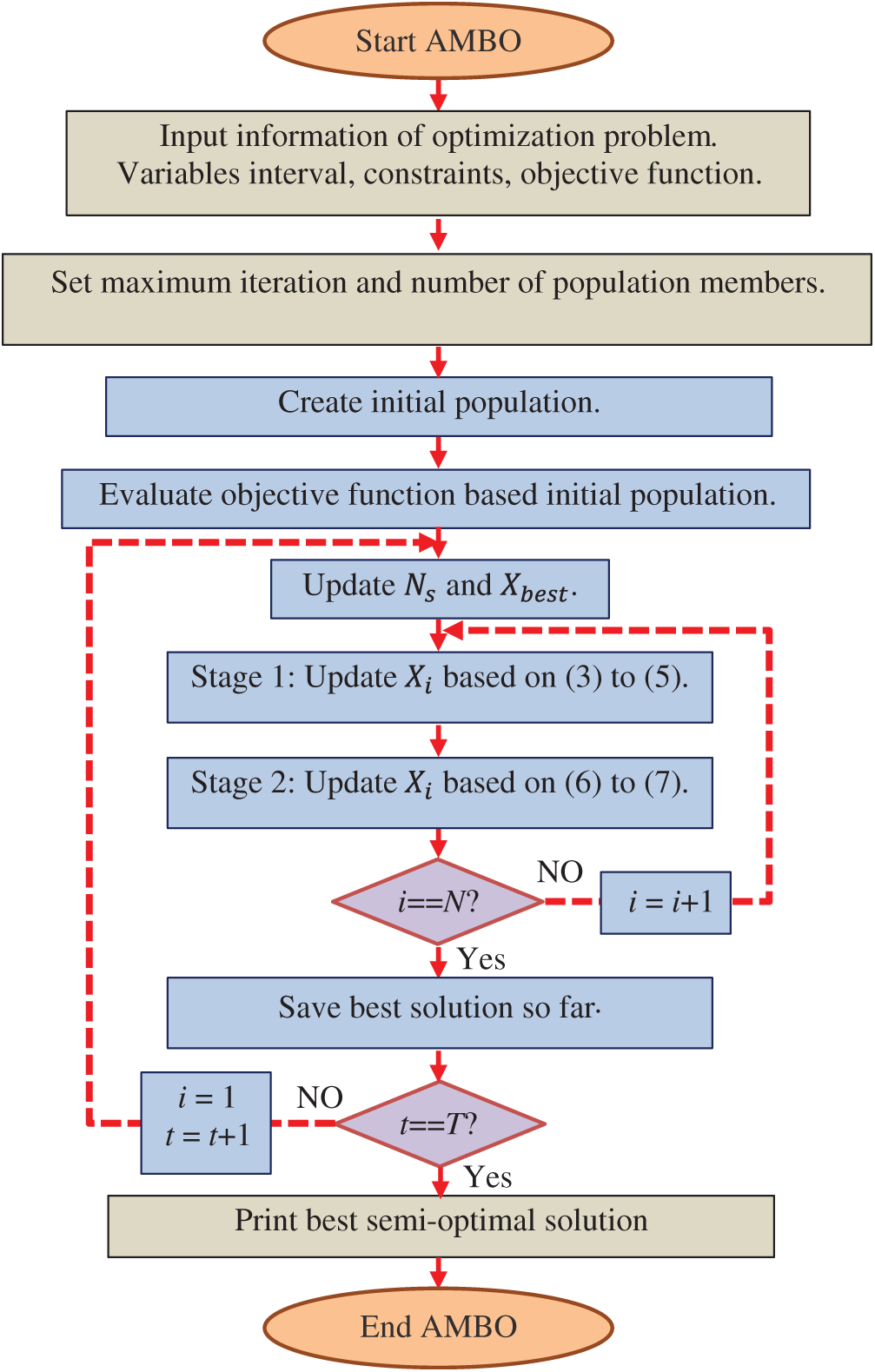Figure 1: Flowchart of AMBO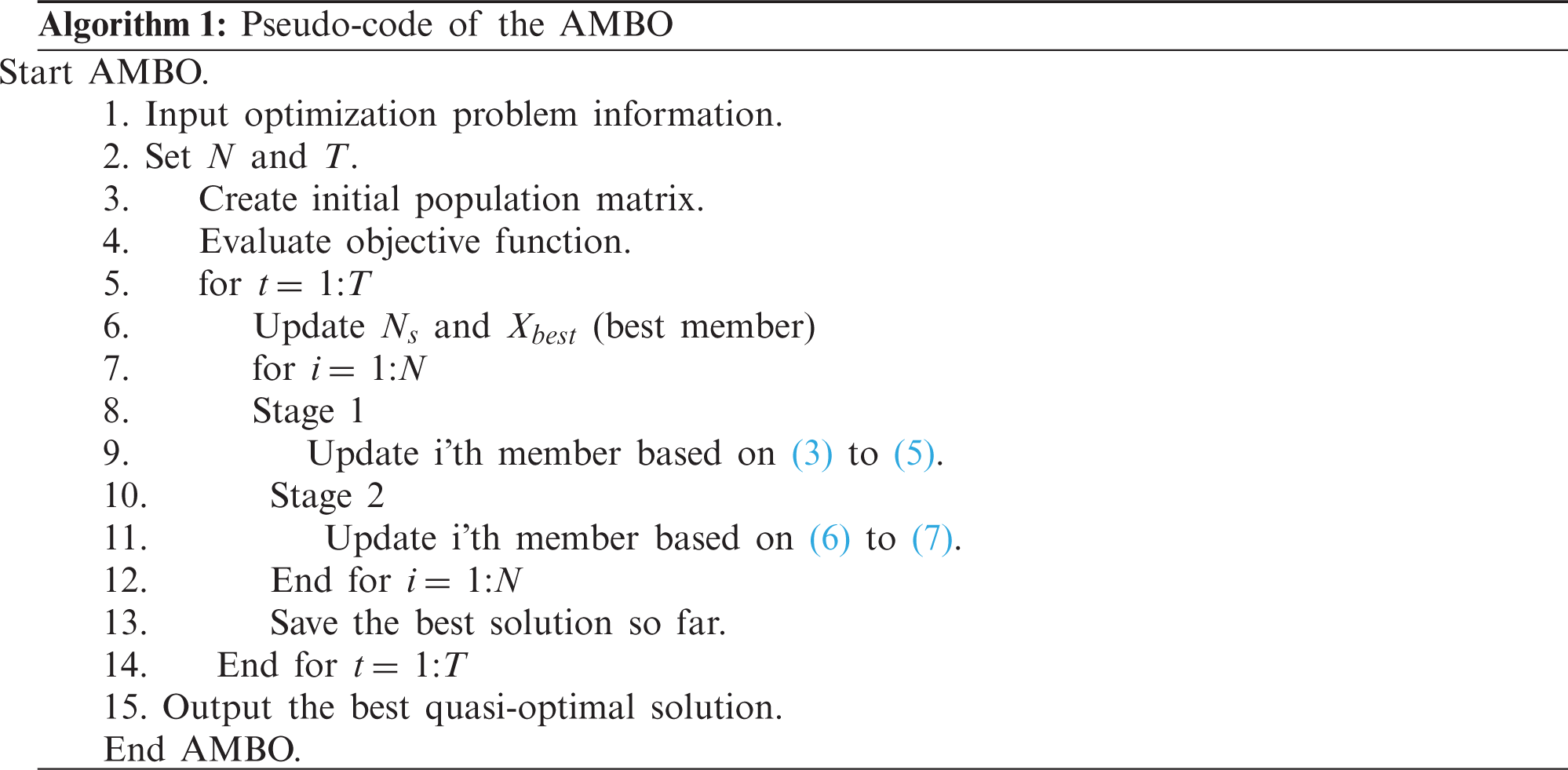3  Simulation Study

In this section, the ability of AMBO for solving optimization problems and providing quasi-optimal solutions is evaluated. For this purpose, AMBO is implemented on a set of twenty-three standard objective functions from three different types including unimodal, high-dimensional multimodal, and fixed-dimensional multimodal functions. Complete information of these objective functions is provided in the Appendix (Tabs. A1 to A3).

3.1 Experimental Setup

In order to analyze the performance of the proposed AMBO in providing the quasi-optimal solution, AMBO is compared with eight other optimization algorithms namely Genetic Algorithm (GA), Particle Swarm Optimization (PSO), Gravitational Search Algorithm (GSA), Teaching Learning-Based Optimization (TLBO), Grey Wolf Optimizer (GWO), Whale Optimization Algorithm (WOA), Marine Predators Algorithm (MPA), and Tunicate Swarm Algorithm (TSA).

To solve each of the objective functions, 20 independent runs of the proposed AMBO has been performed, where each run includes 1000 iterations. The average (Ave) and standard deviation (std) of the best solutions have been used to present the results of optimization for the objective functions.

3.2 Evaluation Results for Objective Functions

The objective functions F1 to F7 belong to unimodal category. These functions have been selected to evaluate the performance of the optimization algorithms. The optimization results for these objective functions using the proposed AMBO and eight other optimization algorithms are presented in Tab. 1. According to this table, AMBO is the best optimizer for F1, F2, F3, F4, and F6 objective functions.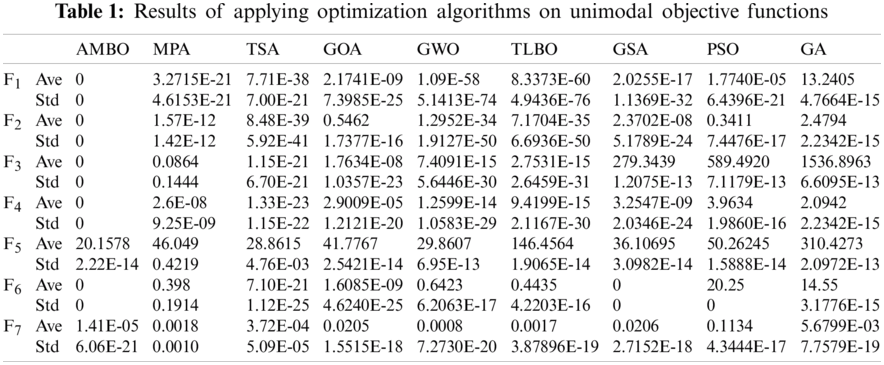Six high-dimensional multimodal objective functions F8 to F13 have been selected to evaluate the performance of the optimization algorithms in providing a suitable quasi-optimal solution. Tab. 2 shows the optimization results for these objective functions using AMBO and eight other optimization algorithms. AMBO is the best optimizer for F8, F9, and F10 objective functions.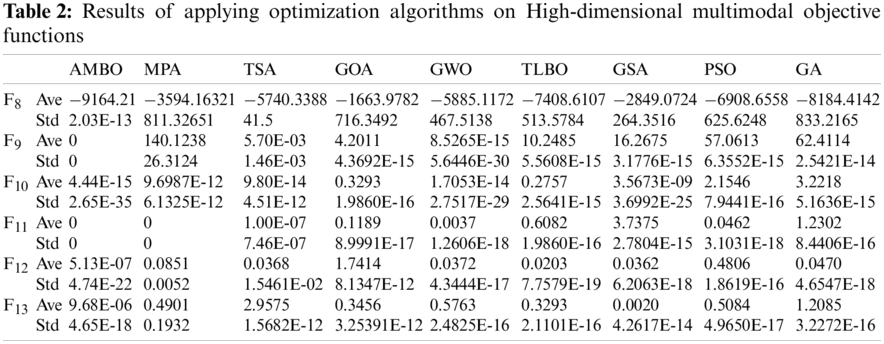The third group of objective functions, including F14 to F23, is selected from the fixed-dimensional multimodal type. The results of the implementation of the proposed algorithm and eight other optimization algorithms on these objective functions are presented in Tab. 3. The results in this table show that AMBO is the best optimizer for F15, F16, and F17 target functions.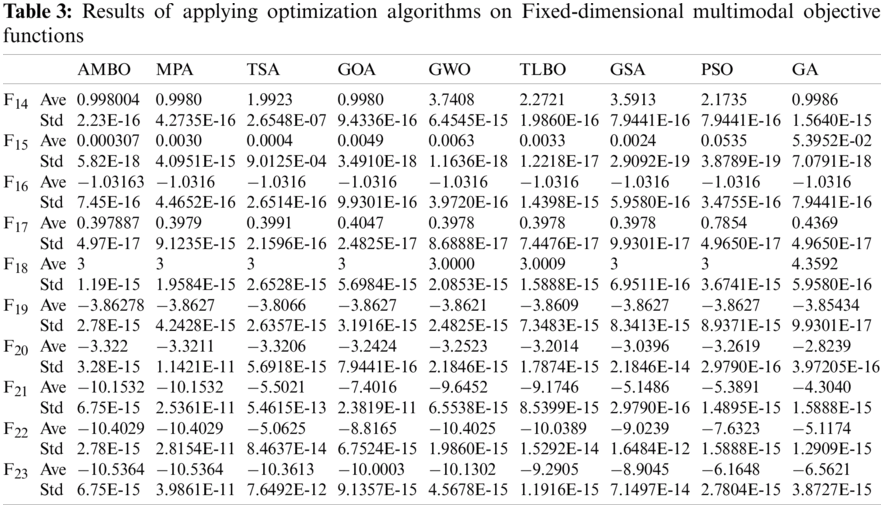3.3 Discussion

Two important indicators for evaluating the performance of the optimization algorithms in solving optimization problems are the exploitation index and the exploration index.

Exploitation index indicates the ability of an optimization algorithm to provide a suitable quasi-optimal solution close to the global optimum for an optimization problem. An optimization algorithm must be able to provide a suitable solution at the end of its iterations. whatever this solution is closer to the global optimum, that algorithm has higher exploitation power. Unimodal objective functions (F1 to F7) have only one optimal solution. Therefore, they are suitable for evaluating the exploitation power of the optimization algorithms. The optimization results of these objective functions show that AMBO has the best performance for all F1 to F7 functions and has higher exploitation power than the other eight optimization algorithms.

Exploration power means the ability of an algorithm to scan the search space properly and accurately. This indicator is especially important for optimization problems that have several local optimal solutions. Therefore, an algorithm that can provide a suitable quasi-optimal solution by accurately scanning the search space and passing through local optimal solutions has high exploration power. The multimodal objective functions of the second and third groups (F8 to F13 and F14 to F23) have several local optimums. Hence, they are suitable for evaluating exploration power. The optimization results of these objective functions presented in Tabs. 3 and 4 indicate the high exploration ability of the proposed AMBO algorithm in solving this type of objective functions.3.4 Sensitivity Analysis

In this section, the sensitivity of the AMBO to the two parameters of maximum number of iteration and number of population members is evaluated.

In order to evaluate the sensitivity of the proposed algorithm to the maximum number of iterations, the AMBO for the maximum number of iterations of 100, 500, 800 and 1000 has been implemented independently on all objective functions. The results of these implementations are presented in Tab. 4. The results show that AMBO converges towards the optimal solution when the number of iterations is increased.

Also, in order to analyze the sensitivity of the proposed algorithm to the number of population members, the AMBO has been implemented independently for different populations with number of 20, 30, 50 and 80 members. The results of this simulation for the number of different members of the population are presented in Tab. 5. It is analyzed from Tab. 5 that the value of fitness function decreases when number of search agents increases.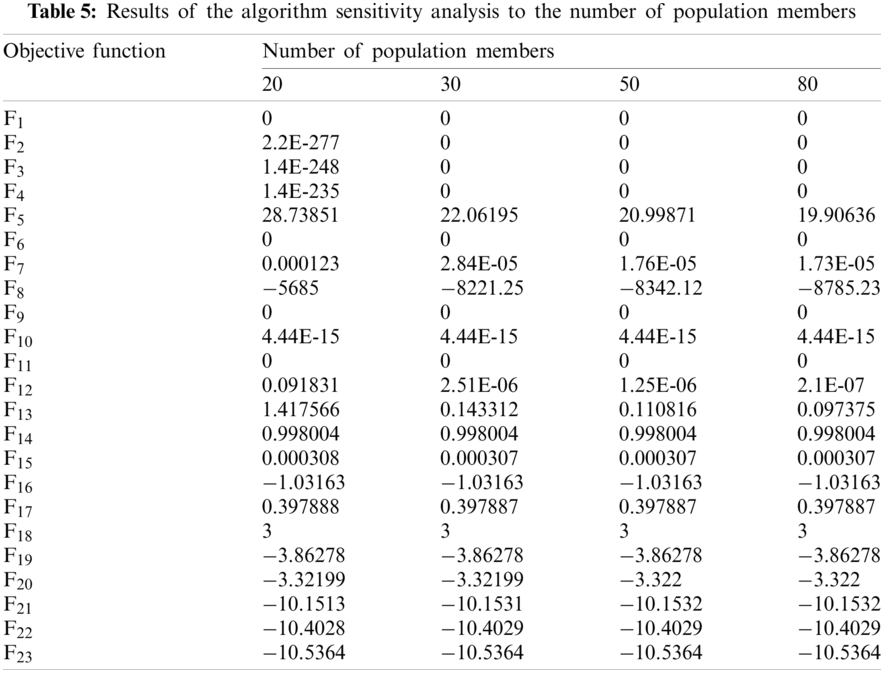4  Statistical Testing

In this section, statistical analysis on the optimization results obtained by different optimization algorithms is presented. Although presenting the results in the form of average and standard deviation provides useful information about the performance of optimization algorithms, statistical analysis of the results is also important for better evaluation. For this purpose, the Wilcoxon rank sum test has been used as a non-parametric statistical test to specify the significance of the results. Wilcoxon rank test is applied to specify whether the results obtained by the proposed AMBO are different from other eight optimization algorithms in a statistically significant way.

A p-value specifies whether the given algorithm is statistically significant or not. If p-value of the given algorithm is less than 0.05, then the corresponding algorithm is statistically significant. The result of analysis using Wilcoxon rank test for the objective functions is shown in Tab. 6. It is observed from Tab. 6 that the p-value obtained from AMBO is much smaller than 0.05 for all the objective functions. Therefore, the proposed AMBO is statistically different from the other competitor algorithms.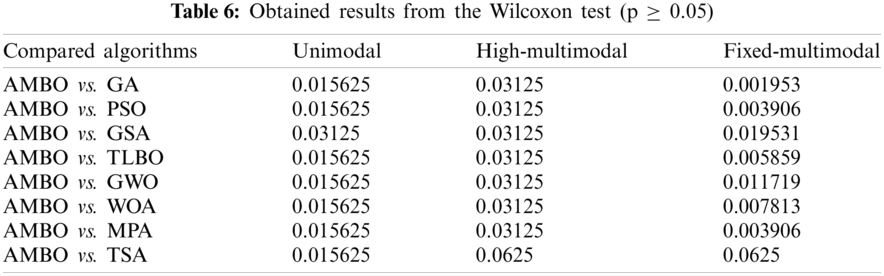5  Conclusions and Future Works

Optimization algorithms are one of the most effective and widely-used methods in solving optimization problems in various fields of science and engineering. In this paper, a new optimization algorithm called All Members-Based Optimizer (AMBO) was presented for solving optimization problems. The proposed AMBO was designed to use more information of different members of the population and to participate all members in updating the algorithm population. AMBO was mathematically modeled and implemented on a set of twenty-three standard objective functions. Also, in order to analyze the results, AMBO was compared with eight optimization algorithms including Genetic Algorithm (GA), Particle Swarm Optimization (PSO), Gravitational Search Algorithm (GSA), Teaching Learning-Based Optimization (TLBO), Grey Wolf Optimizer (GWO), Whale Optimization Algorithm (WOA), Marine Predators Algorithm (MPA), and Tunicate Swarm Algorithm (TSA).

The results of optimizing the unimodal objective functions showed that AMBO is more capable than other algorithms in solving such problems and therefore, it is superior considering the exploitation index. Also, the results of optimization for multimodal objective functions showed that AMBO with high exploration power is able to provide suitable quasi-optimal solutions for this type of functions. Based on the simulation results, it can be concluded that the proposed algorithm has an acceptable ability to solve various optimization problems and is superior and much more competitive than other mentioned optimization algorithms.

The authors suggest some ideas and perspectives for future studies. Design of the binary version as well as the multi-objective version of the AMBO is an interesting potential for future investigations. Apart from this, implementing AMBO on various optimization problems and real-world optimization problems can be considered as some significant contributions, as well.

Funding Statement: PT (corresponding author) was supported by the Excelence project PřF UHK No. 2202/2020-2022 and Long-term development plan of UHK for year 2021, University of Hradec Králové, Czech Republic, https://www.uhk.cz/en/faculty-of-science/about-faculty/official-board/internal-regulations-and-governing-acts/governing-acts/deans-decision/2020#grant-competiti-on-of-fos-uhk-excellence-for-2020.

Conflicts of Interest: The authors declare that they have no conflicts of interest to report regarding the present study.

References

1.  1.  M. Dehghani, Z. Montazeri, A. Dehghani, O. P. Malik, R. Morales-Menendez et al., “Binary spring search algorithm for solving various optimization problems,” Applied Sciences, vol. 11, no. 3, pp. 1286, 2021.
2.  2.  M. Dehghani, Z. Montazeri, A. Dehghani, H. Samet, C. Sotelo et al., “DM: Dehghani method for modifying optimization algorithms,” Applied Sciences, vol. 10, no. 21, pp. 7683, 2020.
3.  3.  M. Dehghani, Z. Montazeri, G. Dhiman, O. Malik, R. Morales-Menendez et al., “A spring search algorithm applied to engineering optimization problems,” Applied Sciences, vol. 10, no. 18, pp. 6173, 2020.
4.  4.  M. Dehghani, Z. Montazeri and O. P. Malik, “Energy commitment: A planning of energy carrier based on energy consumption,” Electrical Engineering & Electromechanics, no. 4, pp. 69–72, 2019.
5.  5.  M. Dehghani, M. Mardaneh, O. P. Malik, J. M. Guerrero, C. Sotelo et al., “Genetic algorithm for energy commitment in a power system supplied by multiple energy carriers,” Sustainability, vol. 12, no. 23, pp. 10053, 2020.
6.  6.  M. Dehghani, M. Mardaneh, O. P. Malik, J. M. Guerrero, R. Morales-Menendez et al., “Energy commitment for a power system supplied by multiple energy carriers system using following optimization algorithm,” Applied Sciences, vol. 10, no. 17, pp. 5862, 2020.
7.  7.  H. Rezk, A. Fathy, M. Aly and M. N. F. Ibrahim, “Energy management control strategy for renewable energy system based on spotted hyena optimizer,” Computers, Materials & Continua, vol. 67, no. 2, pp. 2271–2281, 2021.
8.  8.  A. Ehsanifar, M. Dehghani and M. Allahbakhshi, “Calculating the leakage inductance for transformer inter-turn fault detection using finite element method,” in Proc. of Iranian Conf. on Electrical Engineering, Tehran, Iran, pp. 1372–1377, 2017.
9.  9.  M. Dehghani, Z. Montazeri and O. Malik, “Optimal sizing and placement of capacitor banks and distributed generation in distribution systems using spring search algorithm,” International Journal of Emerging Electric Power Systems, vol. 21, no. 1, pp. 20190217, 2020.
10. 10. M. Dehghani, Z. Montazeri, O. P. Malik, K. Al-Haddad, J. M. Guerrero et al., “A New methodology called dice game optimizer for capacitor placement in distribution systems,” Electrical Engineering & Electromechanics, no. 1, pp. 61–64, 2020.
11. 11. S. Dehbozorgi, A. Ehsanifar, Z. Montazeri, M. Dehghani and A. Seifi, “Line loss reduction and voltage profile improvement in radial distribution networks using battery energy storage system,” in Proc. of IEEE 4th Int. Conf. on Knowledge-Based Engineering and Innovation, Tehran, Iran, pp. 0215–0219, 2017.
12. 12. Z. Montazeri and T. Niknam, “Optimal utilization of electrical energy from power plants based on final energy consumption using gravitational search algorithm,” Electrical Engineering & Electromechanics, no. 4, pp. 70–73, 2018.
13. 13. M. Dehghani, M. Mardaneh, Z. Montazeri, A. Ehsanifar, M. J. Ebadi et al., “Spring search algorithm for simultaneous placement of distributed generation and capacitors,” Electrical Engineering & Electromechanics, no. 6, pp. 68–73, 2018.
14. 14. M. Premkumar, R. Sowmya, P. Jangir, K. S. Nisar and M. Aldhaifallah, “A new metaheuristic optimization algorithms for brushless direct current wheel motor design problem,” Computers, Materials & Continua, vol. 67, no. 2, pp. 2227–2242, 2021.
15. 15. M. Dehghani, Z. Montazeri, A. Ehsanifar, A. R. Seifi, M. J. Ebadi et al., “Planning of energy carriers based on final energy consumption using dynamic programming and particle swarm optimization,” Electrical Engineering & Electromechanics, no. 5, pp. 62–71, 2018.
16. 16. Z. Montazeri and T. Niknam, “Energy carriers management based on energy consumption,” in Proc. of IEEE 4th Int. Conf. on Knowledge-Based Engineering and Innovation, Tehran, Iran, pp. 0539–0543, 2017.
17. 17. J. Kennedy and R. Eberhart, “Particle swarm optimization,” in Proc. of ICNN’95-Int. Conf. on Neural Networks, Perth, WA, Australia, pp. 1942–1948, 1995.
18. 18. M. Dorigo and T. Stützle, “Ant colony optimization: Overview and recent advances,” in Handbook of Metaheuristics, vol. 146, pp. 311–351, 2019.
19. 19. M. Dehghani, M. Mardaneh, J. M. Guerrero, O. P. Malik, R. A. Ramirez-Mendoza et al., “A new “doctor and patient” optimization algorithm: An application to energy commitment problem,” Applied Sciences, vol. 10, no. 17, pp. 5791, 2020.
20. 20. G. Dhiman, K. K. Singh, M. Soni, A. Nagar, M. Dehghani et al., “MOSOA: A new multi-objective seagull optimization algorithm,” Expert Systems with Applications, vol. 167, pp. 114150, 2020.
21. 21. S. Mirjalili and A. Lewis, “The whale optimization algorithm,” Advances in Engineering Software, vol. 95, pp. 51–67, 2016.
22. 22. X.-S. Yang, “Firefly algorithm, stochastic test functions and design optimization,” Arxiv, vol. 2, no. 2, pp. 78–84, 2010.
23. 23. D. Karaboga and B. Basturk, “Artificial bee colony (ABC) optimization algorithm for solving constrained optimization problems,” In: P. Melin, O. Castillo, L. T. Aguilar, J. Kacprzyk, and W. Pedrycz (eds.Foundations of Fuzzy Logic and Soft Computing. IFSA 2007. Lecture Notes in Computer Science, vol. 4529, Berlin, Heidelberg: Springer, 2007. https://doi.org/10.1007/978-3-540-72950-1_77.
24. 24. A. H. Gandomi, X.-S. Yang and A. H. Alavi, “Cuckoo search algorithm: A metaheuristic approach to solve structural optimization problems,” Engineering with Computers, vol. 29, no. 1, pp. 17–35, 2013.
25. 25. X. S. Yang, “A new metaheuristic bat-inspired algorithm,” In: J. R. González, D. A. Pelta, C. Cruz, G. Terrazas, and N. Krasnogor (eds.Nature Inspired Cooperative Strategies for Optimization (NICSO 2010). Studies in Computational Intelligence, vol. 284, Berlin, Heidelberg: Springer, 2010. https://doi.org/10.1007/978-3-642-12538-6_6.
26. 26. G. Dhiman and V. Kumar, “Spotted hyena optimizer: A novel bio-inspired based metaheuristic technique for engineering applications,” Advances in Engineering Software, vol. 114, pp. 48–70, 2017.
27. 27. A. Mucherino and O. Seref, “Monkey search: A novel metaheuristic search for global optimization,” in AIP Conf. Proc. American Institute of Physics, vol. 953, no. 1, pp. 162–173, 2007.
28. 28. M. Neshat, G. Sepidnam, M. Sargolzaei and A. N. Toosi, “Artificial fish swarm algorithm: A survey of the state-of-the-art, hybridization, combinatorial and indicative applications,” Artificial Intelligence Review, vol. 42, no. 4, pp. 965–997, 2014.
29. 29. M. Dehghani, Z. Montazeri, A. Dehghani, and O. P. Malik, “GO: Group optimization,” Gazi University Journal of Science, vol. 33, pp. 381–392, 2020.
30. 30. Y. Shiqin, J. Jianjun and Y. Guangxing, “A dolphin partner optimization,” in 2009 WRI Global Congress on Intelligent Systems, Xiamen, China, vol. 1, pp. 124–128, 2009.
31. 31. R. Oftadeh, M. Mahjoob and M. Shariatpanahi, “A novel meta-heuristic optimization algorithm inspired by group hunting of animals: Hunting search,” Computers & Mathematics with Applications, vol. 60, no. 7, pp. 2087–2098, 2010.
32. 32. H. Zhang and Q. Hui, “A coupled spring forced bat searching algorithm: Design, analysis and evaluation,” in 2020 American Control Conf., Denver, CO, USA, pp. 5016–5021, 2020.
33. 33. R. V. Rao, V. J. Savsani and D. Vakharia, “Teaching–learning-based optimization: A novel method for constrained mechanical design optimization problems,” Computer-Aided Design, vol. 43, no. 3, pp. 303–315, 2011.
34. 34. S. Mirjalili, S. M. Mirjalili and A. Lewis, “Grey wolf optimizer,” Advances in Engineering Software, vol. 69, pp. 46–61, 2014.
35. 35. M. Dehghani, M. Mardaneh and O. Malik, “FOA: ‘Following’ optimization algorithm for solving power engineering optimization problems,” Journal of Operation and Automation in Power Engineering, vol. 8, no. 1, pp. 57–64, 2020.
36. 36. S. Mirjalili, “Moth-flame optimization algorithm: A novel nature-inspired heuristic paradigm,” Knowledge-Based Systems, vol. 89, pp. 228–249, 2015.
37. 37. S. Saremi, S. Mirjalili and A. Lewis, “Grasshopper optimisation algorithm: Theory and application,” Advances in Engineering Software, vol. 105, pp. 30–47, 2017.
38. 38. M. Dehghani, M. Mardaneh, O. P. Malik and S. M. NouraeiPour, “DTO: Donkey theorem optimization,” in Proc. of Iranian Conf. on Electrical Engineering, Yazd, Iran, pp. 1855–1859, 2019.
39. 39. G. Dhiman and V. Kumar, “Emperor penguin optimizer: A bio-inspired algorithm for engineering problems,” Knowledge-Based Systems, vol. 159, pp. 20–50, 2018.
40. 40. M. Dehghani, Z. Montazeri, A. Dehghani, R. R. Mendoza, H. Samet et al., “MLO: Multi leader optimizer,” International Journal of Intelligent Engineering and Systems, vol. 13, no. 6, pp. 364–373, 2020.
41. 41. G. Dhiman, M. Garg, A. K. Nagar, V. Kumar and M. Dehghani, “A novel algorithm for global optimization: Rat swarm optimizer,” Journal of Ambient Intelligence and Humanized Computing, 2020. https://doi.org/10.1007/s12652-020-02580-0.
42. 42. H. Givi, M. Dehghani, Z. Montazeri, R. Morales-Menendez, R. A. Ramirez-Mendoza et al., “GBUO: The good, the bad, and the ugly, optimizer,” Applied Sciences, vol. 11, no. 5, pp. 2042, 2021.
43. 43. M. Dehghani, Z. Montazeri, A. Dehghani and A. Seifi, “Spring search algorithm: A new meta-heuristic optimization algorithm inspired by hooke’s law,” in Proc. of IEEE 4th Int. Conf. on Knowledge-Based Engineering and Innovation, Tehran, Iran, pp. 0210–0214, 2017.
44. 44. M. Dehghani and H. Samet, “Momentum search algorithm: A new meta-heuristic optimization algorithm inspired by momentum conservation law,” SN Applied Sciences, vol. 2, no. 10, pp. 1–15, 2020.
45. 45. E. Rashedi, H. Nezamabadi-Pour and S. Saryazdi, “GSA: A gravitational search algorithm,” Information Sciences, vol. 179, no. 13, pp. 2232–2248, 2009.
46. 46. O. K. Erol and I. Eksin, “A new optimization method: Big bang–big crunch,” Advances in Engineering Software, vol. 37, no. 2, pp. 106–111, 2006.
47. 47. H. Shah-Hosseini, “Principal components analysis by the galaxy-based search algorithm: A novel metaheuristic for continuous optimisation,” International Journal of Computational Science and Engineering, vol. 6, no. 1–2, pp. 132–140, 2011.
48. 48. A. Kaveh and S. Talatahari, “A novel heuristic optimization method: Charged system search,” Acta Mechanica, vol. 213, no. 3–4, pp. 267–289, 2010.
49. 49. W. F. Sacco and C. Oliveira, “A new stochastic optimization algorithm based on a particle collision metaheuristic,” in 6th World Congresses of Structural and Multidisciplinary Optimization, Rio de Janeiro, Brazil, 30 May–03 June, 2005.
50. 50. P. J. Van Laarhoven and E. H. Aarts, “Simulated Annealing,” in Simulated Annealing: Theory and Applications, Dordrecht, Netherlands: Springer, pp. 7–15, 1987.
51. 51. M. Dehghani, Z. Montazeri, A. Dehghani, N. Nouri and A. Seifi, “BSSA: Binary spring search algorithm,” in Proc. of IEEE 4th Int. Conf. on Knowledge-Based Engineering and Innovation, Tehran, Iran, pp. 0220–0224, 2017.
52. 52. R. A. Formato, “Central force optimization: A new nature inspired computational framework for multidimensional search and optimization,” Nature Inspired Cooperative Strategies for Optimization, Berlin, Heidelberg: Springer, pp. 221–238, 2008.
53. 53. A. Kaveh and M. Khayatazad, “A new meta-heuristic method: Ray optimization,” Computers & Structures, vol. 112, pp. 283–294, 2012.
54. 54. F. F. Moghaddam, R. F. Moghaddam and M. Cheriet, “Curved space optimization: A random search based on general relativity theory,” Arxiv Preprint Arxiv: 1208.2214, 2012.
55. 55. F. A. Hashim, E. H. Houssein, M. S. Mabrouk, W. Al-Atabany and S. Mirjalili, “Henry gas solubility optimization: A novel physics-based algorithm,” Future Generation Computer Systems, vol. 101, pp. 646–667, 2019.
56. 56. H. Du, X. Wu and J. Zhuang, “Small-world optimization algorithm for function optimization,” in Proc. of Int. Conf. on Natural Computation, Berlin, Heidelberg, pp. 264–273, 2006.
57. 57. B. Alatas, “ACROA: Artificial chemical reaction optimization algorithm for global optimization,” Expert Systems with Applications, vol. 38, no. 10, pp. 13170–13180, 2011.
58. 58. A. Bose, T. Biswas and P. Kuila, “A novel genetic algorithm based scheduling for multi-core systems,” in Smart Innovations in Communication and Computational Sciences, Singapore, Springer, pp. 45–54, 2019.
59. 59. S. A. Hofmeyr and S. Forrest, “Architecture for an artificial immune system,” Evolutionary Computation, vol. 8, no. 4, pp. 443–473, 2000.
60. 60. L. J. Fogel, A. J. Owens and M. J. Walsh, “Artificial intelligence through simulated evolution,” in Evolutionary Computation: The Fossil Record, IEEE, pp. 227–296, 1998. https://doi.org/10.1109/9780470544600.ch7.
61. 61. R. G. Reynolds, “An introduction to cultural algorithms,” in Proc. of the third Annual Conf. on Evolutionary Programming, river edge, NJ: World Scientific, vol. 24, pp. 131–139, 1994.
62. 62. H.-G. Beyer and H.-P. Schwefel, “Evolution strategies–a comprehensive introduction,” Natural Computing, vol. 1, no. 1, pp. 3–52, 2002.
63. 63. S. Das and P. N. Suganthan, “Differential evolution: A survey of the state-of-the-art,” IEEE Transactions on Evolutionary Computation, vol. 15, no. 1, pp. 4–31, 2011.
64. 64. D. Simon, “Biogeography-based optimization,” IEEE Transactions on Evolutionary Computation, vol. 12, no. 6, pp. 702–713, 2008.
65. 65. G. Huang, “Artificial infectious disease optimization: A SEIQR epidemic dynamic model-based function optimization algorithm,” Swarm and Evolutionary Computation, vol. 27, pp. 31–67, 2016.
66. 66. J. R. Koza, “Genetic programming as a means for programming computers by natural selection,” Statistics and Computing, vol. 4, no. 2, pp. 87–112, 1994.
67. 67. W. Deng, H. Liu, J. Xu, H. Zhao and Y. Song, “An improved quantum-inspired differential evolution algorithm for deep belief network,” IEEE Transactions on Instrumentation and Measurement, vol. 69, no. 10, pp. 7319–7327, 2020.
68. 68. M. Dehghani, M. Mardaneh, J. M. Guerrero, O. Malik and V. Kumar, “Football game based optimization: An application to solve energy commitment problem,” International Journal of Intelligent Engineering and Systems, vol. 13, pp. 514–523, 2020.
69. 69. M. Dehghani, Z. Montazeri, H. Givi, J. M. Guerrero and G. Dhiman, “Darts game optimizer: A new optimization technique based on darts game,” International Journal of Intelligent Engineering and Systems, vol. 13, pp. 286–294, 2020.
70. 70. M. Dehghani, Z. Montazeri, O. P. Malik, A. Ehsanifar and A. Dehghani, “OSA: Orientation search algorithm,” international journal of industrial electronics,” Control and Optimization, vol. 2, no. 2, pp. 99–112, 2019.
71. 71. M. Dehghani, Z. Montazeri, S. Saremi, A. Dehghani, O. P. Malik et al., “HOGO: Hide objects game optimization,” International Journal of Intelligent Engineering and Systems, vol. 13, no. 4, pp. 216–225, 2020.
72. 72. D. Mohammad, M. Zeinab, O. P. Malik, H. Givi and J. M. Guerrero, “Shell game optimization: A novel game-based algorithm,” International Journal of Intelligent Engineering and Systems, vol. 13, no. 3, pp. 246–255, 2020.
73. 73. M. Dehghani, Z. Montazeri, O. P. Malik, G. Dhiman and V. Kumar, “BOSA: Binary orientation search algorithm,” International Journal of Innovative Technology and Exploring Engineering, vol. 9, no. 1, pp. 5306–5310, 2019.
74. 74. M. Dehghani, Z. Montazeri and O. P. Malik, “DGO: Dice game optimizer,” Gazi University Journal of Science, vol. 32, no. 3, pp. 871–882, 2019.

Appendix A. Information of objective function

Information on the twenty-three objective functions used in the simulations is presented in Tabs. A1 to A3.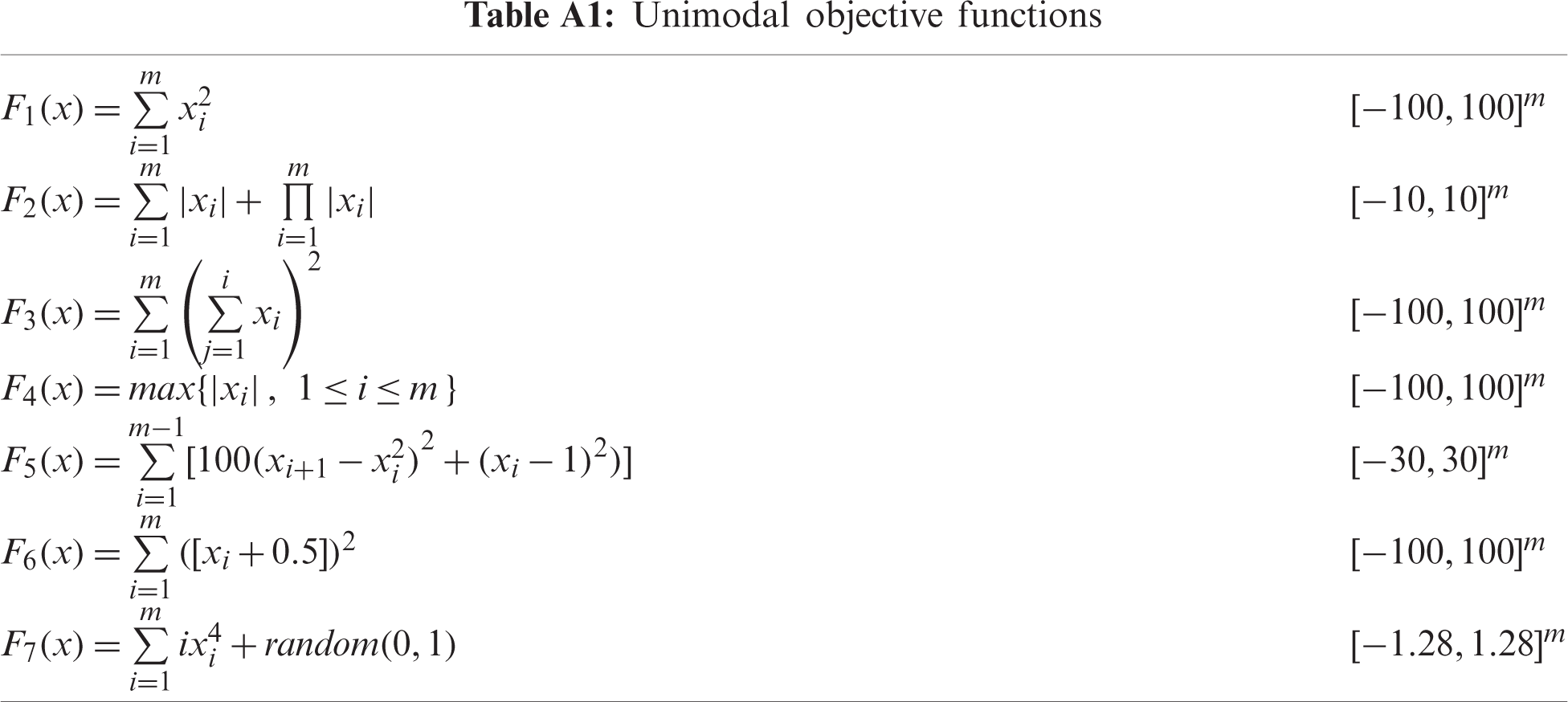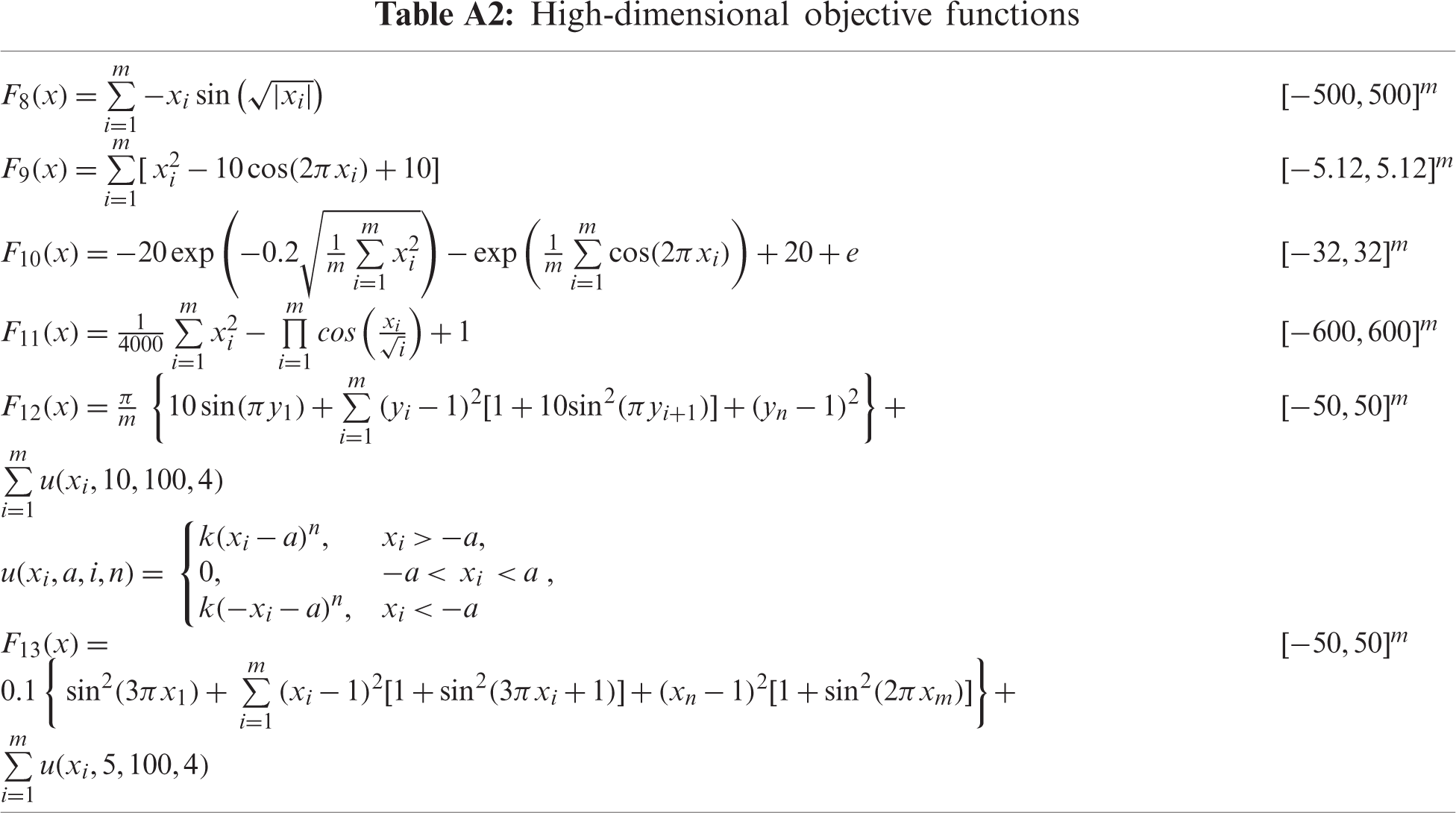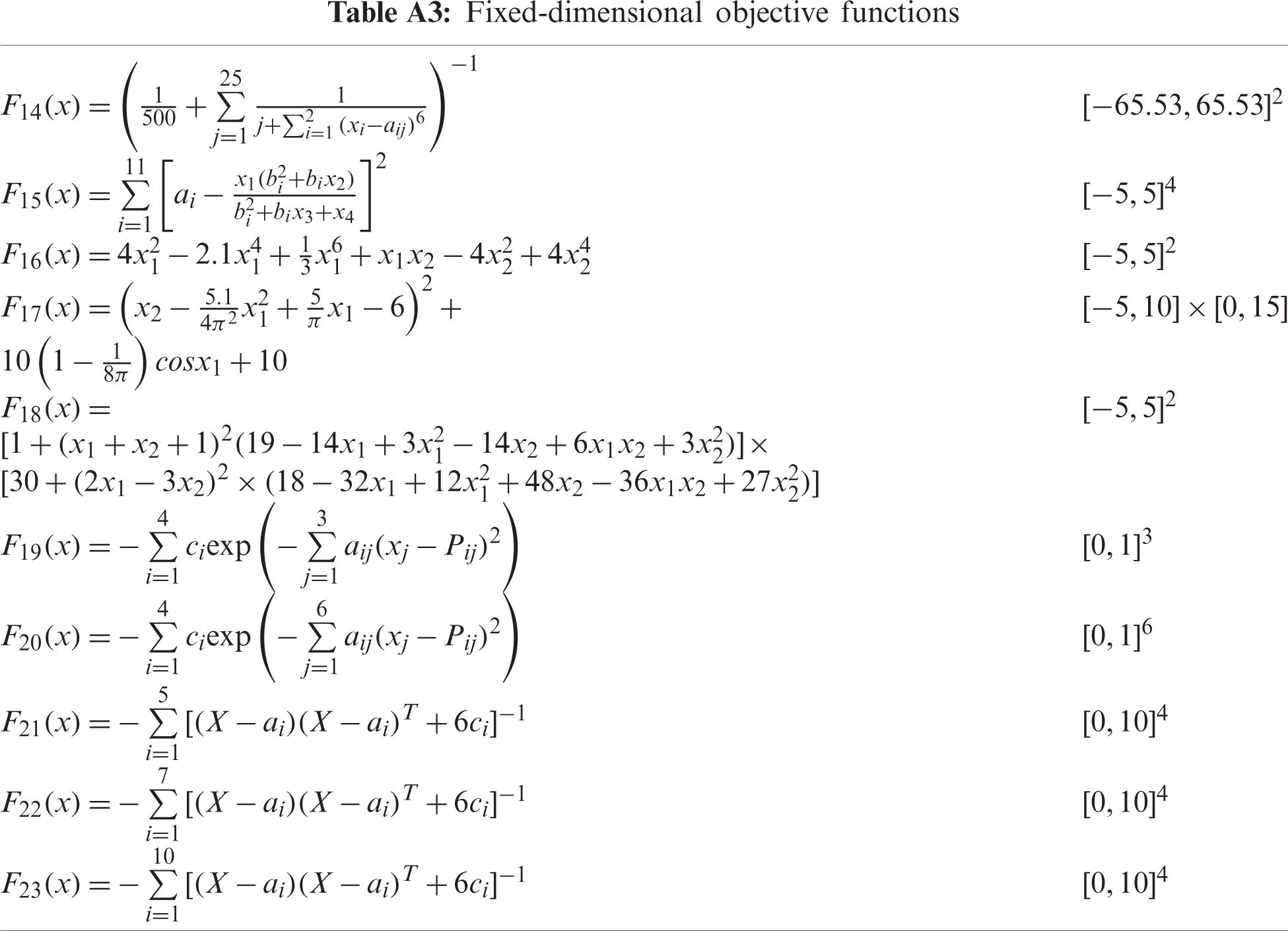This work is licensed under a Creative Commons Attribution 4.0 International License, which permits unrestricted use, distribution, and reproduction in any medium, provided the original work is properly cited.